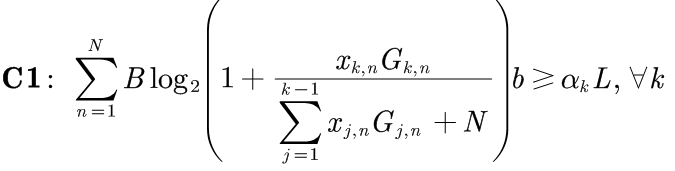# How to solve this problem?Cannot perform the operation: {real affine} .* {convex}

My MATLAB code is shown below:The error is :
Disciplined convex programming error:
Cannot perform the operation: {real affine} .* {convex}

Would anyone give me some idea about it?

Have you proven this is a convex optimization problem?

Thanks a lot, Mark, but I’ve read it before and I found one of the constraints is concave function with respect to the variable, and the following is the optimization problem, where F(X)={0,1},if X is true then, F(X)=1 and F(X)=0, otherwise.
And how can I transform it to convex problem? Thanks very much.

How have you proved the convexity of C2?

Perhaps Can CVX solve this kind of function {x-log(1+0.01*x/(x+1))} will help you for C2. I haven’t worked out whether modifications to handle the sum in the denominator inside the log work out. But the formulation in the link would work if there were not a sum in that denominator.

Looking at it again, I am paying attention to the x’s having different indices in each term of the sum in the denominator of the log, which therefore makes the whole thing look neither convex nor concave.

if you have proof showing the convexity of the constraint (concavity of LHS), please show us.

Sorry, Mark, maybe I should explain it a little bit more clearly. In my model, there are only two elements in set Sn, so the sum in the denominator only has one element. For example, set S1 has G1,1 and G2,1, and G1,1<G2,1, then the sum in the denominator changes into x1,1*G1,1.

That is not clear to me. If there is a non-zero term in the denominator which has j \ne k, then I don’t believe the constraint will be convex. If it only has j = k, then you can use the formulation in the link.

The paper I referred to says it’s convex, and the link to the paper: https://ieeexplore.ieee.org/document/8891923

Sorry, Mark, the following is another link to the paper, and it says the optimization problem is convex in section 3.B (Energy-Efficient Power Optimiaztion). Moreover, I think the problem I’ve posted is convex.
http://geokarag.webpages.auth.gr/wp-content/papercite-data/pdf/j272.pdf

Exactly where in that paper is constraint C1, not to mention proof of its convexity?

Actually, constraint C1 can be rewritten as:where the left-hand side of the inequality is a concave function with respect to x, and the objective function is a linear function w.r.t x, therefore, this is a convex optimization problem. All above is what I understand.

You claim the LHS of C1 s concave w.r.t. x. Please show is the proof.

Here is a simple counterexample, pet my interpretation.

Consider `f(x,y) = log2(1+y/(x+1))` . Its Hessian w.r.t x and y evaluated at `x=y=1` has one negative and one positive eigenvalue. Hence, `f` is neither concave nor convex. How is `f` not a special case of the LHS of C1?

C1 has the same form as constraint C2 of question 28 in the paper. What I think is that, when focus on x_{k,n}, the other variables except x_{k,n} can be treated as constants, since there’s no x_{k,n} in the denominator, the LHS of C1 is a concave function of x_{k,n}. I’m very confused right now…

Convexity in optimization means joint convexity in all the optimization variables. If you fix one or more of the variables, that is a different problem.

in any event, it is your project, .so I’ll let you figure it out. I will encourage you once again to carefully read

I have no reason to believe you will find CVX useful for this problem. Of course you can always fix enough varaibles and produce a nice problem CVX can handle. But then you are left with the need for some iterative scheme around that.

Thank you, I will analyze my optimization problem carefully and find an appropriate solution for it.